## Articulo

•Citado por SciELO
•Accesos

•Similares en SciELO

## versión On-line ISSN 1390-6542versión impresa ISSN 1390-9363

### Enfoque UTE vol.9 no.4 Quito oct./dic. 2018

#### http://dx.doi.org/10.29019/enfoqueute.v9n4.394

Automatización y Control,…

Predictive ADRC control using LMI

Control ADRC Predictivo utilizando LMI

1 Universidad Nacional Experimental Rafael María Baralt, Ciudad Ojeda- Venezuela Hanna2k2@gmail.com.

Abstract:

On recent times Active Disturbance Rejection Control has drawn both the attention of industrial and scientific community due to its capability to handle uncertainties and nonlinearities without using an explicit model of the system; on this paper a modification of the ADRC algorithm is presented, first an Extended State Predictor is calculated and later, a controller is built using the LMI approach, the proposal is evaluated through simulations on the Cayley-Rodriguez representation of the Monoaxial satellite.

Keywords: State Estimation; Linear Matrix Inequalities; Process Control; Digital Control; Kalman Filtering

Resumen:

En tiempos recientes, el Control Activo por Rechazo de Perturbaciones (o ADRC por sus siglas en Ingles) ha despertado el interés tanto de la comunidad científica como industrial debido a su capacidad de manejar incertidumbres y no linealidades sin necesitar un modelo explícito del sistema. En este trabajo se presenta una modificación del algoritmo ADRC, primero se construye un Predictor de Estados Extendido para luego, construir el controlador utilizando las Desigualdades Lineales Matriciales, la propuesta es evaluada a través de simulaciones sobre la representación del satélite monoaxial de Cayley Rodriguez.

Palabras clave: Estimación de Estados; Desigualdades Lineales Matriciales; Control de Procesos; Control Digital; Filtro de Kalman

1. Introduction

One of the main concerns in control systems is to find a suitable model for control design, from classical to modern control theory, finding a model that could represent the increasing complexity and nonlinearities of systems is a difficult step to be fulfilled in order to find a stabilizing controller for a given systems, this need leads to a shift on the control paradigm, (Gao 2006).

The main idea behind Active Disturbance rejection control or ADRC is to build a control law based on Active estimation and rejection of the generalized disturbance using an Extended State Observer or ESO to estimate the internal plant dynamic and external disturbances requiring little information of the plant, (Zheng 2009). This method has been tested in several applications, from process found on laboratories to complex aerospace systems as seen on: (Teppa et al 2012), (Zheng 2009), (Zheng et al 2010), (Alexander et al 2008) and (Tanaka et al 2015).

On this paper, the Extended State Observer is transformed into an Extended State Predictor; taking as starting point the works of (Miklosovic et al 2006) and (Tanaka et al 2015) a Kalman filter in prediction mode is built, this done to deal not only with the unknown dynamics and disturbances but also unknowns time delays. On the other hand, the controller is obtained by using the LMI approach as systematic way to tune the controller not only when the dynamic of the plant is unknown but also if the process is presented in polytopic form.

The paper is structured as follows; first the Extended State Predictor is presented followed by the controller design using the LMI approach to finally test on a benchmark process which in this case is the Cayley-Rodriguez representation of the Monoaxial satellite.

2. Methodology

2.1. Extended State Predictor

First, consider a nonlinear plant described by the differential Equation 1.

The design procedure of the Predictive ADRC controller proposed on this work is divided in two steps following (Gao 2006) ; the first step comprehend the design of an Extended State Predictor (ESP) using (Miklosovic et al 2006) as starting point, the second step involves the design of the corresponding controller using the parameters estimated by the ESP.

In order to build the corresponding ESP, consider the unknown second order transfer function in Equation 2.

this can be rewritten as in Equation 3.

Using the auxiliary representation presented on (Gao 2006) and (Teppa et al 2012) we have Equation 4.

This is written in the state space representation in Equation 5.

Equation 5 is discretized assuming a non-pathological sampling period T according to (Miklosovic et al 2006) using (Astrom et al 1998), as in Equation 6.

Where (Equation 7):

According to Equation 7, Equation 5 becomes Equation 8.

On this work is proposed to use Equation 8 with a Kalman Filter on prediction mode, for this reason, consider (Astrom et al 1998) where the system is presented as a one step ahead predictor of the form in Equation 9.

The value of K E is obtained by solving the Ricatti Equation, (Astrom et al 1998), (Tanaka et al 2015) as in Equation 10.

The gain of the predictor is described by Equation 11.

2.2. Controller Design

Once the Extended State Predictor is designed, the following step is to build the corresponding controller. Consider a reduced representation of Equation 8, which is controllable and observable as in Equation 12.

On this work b 0 is considered as part of the system to be controlled, on (Gao 2006) and (Teppa et al 2012) a pole placement design method is used as starting point, whereas this work proposes the use of an LMI in discrete time.

According to (Kaskurewicz et al 2006), (Xue et al 2009), (Teppa 2012), (Oishi 2008), (Hermann 2006) and (Alamo 2012) consider the Lyapunov equation on discrete time (Equation 13).

Find a desirable control law that minimizes the following cost function (Equation 14).

First, the difference operator is applied on Equation 13; this is shown in Equation 15.

Where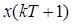is represented by Equation 12 and we have Equation 16.

Substituting Equation 12 and Equation 16 into Equation 15, the Equation 17 is obtained.

Rewriting Equation 17 it is possible to find the control law that stabilizes the system on Equation 12, by solving the Linear Matrix Inequality in Equation 18, with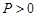, (Stoorvorgel et al 1998).

Where the control law is expressed in Equation 19.

From the values of Equation 19, the parameters of the ADRC controller are obtained according to (Zheng 2009) adapted for discrete time on this work from (Jantzen 1998), this leads to Equation 20.

The Predictive ADRC controller is represented in Figure 1.

3. Case Study

In order to study the Predictive ADRC controller presented before, the Cayley-Rodriguez representation of the Monoaxial satellite found in (Sira et al 2005) and (Andymar 2006) is selected, this is a nonlinear plant with unstable linear models in any equilibrium point, and is represented by Equation 21.

Where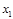is the orientation angle of the satellite respect of an oblique’s axis and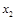is the angular speed respect the principal axis, J is the inertia and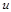is the applied torque, as seen in Figure 2 (Sira et al 2005).

The first step is to build the discrete time representation of the unknown system, assuming a sampling period of T=0.1 seg and considering b 0 =0.01 substituting on Equation 8; the unknown model is shown in Equation 22.

Using Equation 22, the gain of the predictor K E is shown in Equation 23.

Once build the ESP, the next step is to build the controller, by choosing Equation 24.

And the reduced Equation 12 can be seen in Equation 25.

With all this on mind and solving for Equation 18 the control law that minimizes Equation 14, we have Equation 26.

The Matlab/Simulink toolboxes where used for all the calculations. On (Sira et al 2005) the Output of the system is x 1 and its desired reference is x 1 =1.5 with J=90 (Andymar 2006), the simulation is presented in Figure 3 against a disturbance of the 50% of the nominal value.

As shown on Figure 3, the Predictive ADRC effectively regulates the system and also has the capability to reject larger disturbances.

On the second experiment, the discrete nominal linearized model of Equation 22 is used, assuming x 1 =1.5 and x 2 =0 as equilibrium points, the discrete linearized model with sampling period T=0.1seg is presented in Equation 27.

The ESP presented before on Equation 22 and Equation 23 is used, so this experiment is limited to the control law calculation, on this case assuming Equation 28.

The controller is shown in Equation 29.

Against the same reference signal and disturbance, the system is shown in Figure 4.

The response is improved when the overshoot is reduced; this is because the controller has more information of the system than the unknown model presented before.

On both cases including b 0 =0.01 into the control calculation and eliminating from the block diagram as presented on Equation 20 and Figure 1; leads to a reduction on the sensibility on the control loop, mostly against measurable and not measurable disturbances.

4. Conclusions

On this paper a modification of the typical ADRC algorithm is presented, first an ESO is used as a predictive estimator that feeds the ADRC controller on discrete time, which is tuned using the LMI´s approach, resulting on a controller who can reject disturbance and track the desired reference, on future works a physical implementation of this controller is considered on test system.

References

Stoorvorgel, A., and Saberi, A. (1998). “The Discrete Algebraic Ricatti Equation and Linear Matrix Inequalities”. Linear Algebra and Its Applications 274:317-365. [ Links ]

B, A., Rarick, R., and Dong, L. (2008). “A novel application of an extended State Observer for High Performance control of NASA’s HSS Flywheel and Fault Detection” Proceeding American Control Conference 2008, Seattle, Washington, EUA. [ Links ]

Xue, D., & Chen, Y. (2009). Solving applied mathematical problems with Matlab CRC Press. [ Links ]

Kaszkurewicz, E., and Bhaya, A. (2006). Matrix Diagonal Stability in Systems and Computation, Birkhauser. [ Links ]

Hermann, G. (2006). "Linear Matrix Inequalities in Control", Lecture Notes, Leicester University. [ Links ]

Ramires, H., Marquez, R., Rivas, F., and Llanes, O. (2005). Control de Sistemas Nolineales, Pearson [ Links ]

Jantzen, j. (1998) “Tunning of Fuzzy PID controllers” Technical University of Denmark, Department of Automation, Lyngby, Denmark. [ Links ]

Astrom, K., and Wittenmark, B.(1998). Computer Controlled System: Theory and Design, Prentice Hall. [ Links ]

Oishi, M.(2008). "Global quadratic lyapunov function", Lecture Notes, University of British Columbia. [ Links ]

Teppa, P. (2012). "Notas en control por desigualdades lineales matriciales", Universidad Simón Bolívar. [ Links ]

Teppa, P., Bravo, M., and Garcia, G. (2012). "Control por rechazo activo de perturbaciones del nivel de líquido de un sistema de tanques acoplado" Revista Faraute de Ciencia y Tecnología, Vol 7, No 1,10-18. [ Links ]

Zheng, Q., and Gao, Z. (2010). “On Practical Aplications of Active Disturbance Rejection Control”. Proceedings of the 29 th Chinese Control Conference, Beijing .China.. [ Links ]

Zheng, Q. ((2009). ”On Active Disturbance Rejection Control Stability Analysis and aplications in disturbance decoupling control”. Phd Thesis, Cleveland State University. [ Links ]

Andymar, R.(2006). "Uso de GNU Octave para simulacion de sistemas Nolineales", Tesis de Grado ULA. [ Links ]

Miklosovic, R., and Gao, Z. (2006). "Discrete Implementation and generalization of the extended State Observer", Proceeding American Control Conference 2006, Minneapolis, Mn, EUA. [ Links ]

Tanaka, R., Murakami, T., and Ishida, Y. (2015) “Model Matching Control Based on Discrete time improved ADRC considering plant input disturbances”. 2015 Third International Conferences on Artificial Intelligence, Modelling and Simulation. Kota Kinabalu, Sabah, Malaysia. [ Links ]

Alamo-Cantarero, T.(2012). “Minicurso LMI”, Lecture Notes , UNED. [ Links ]

Gao, Z. (2006). "Active Disturbance rejection Control a paradigm Shift in System Control Design" Proceeding American Control Conference 2006, Minneapolis, Mn, EUA [ Links ]

Received: May 15, 2018; Accepted: October 30, 2018This is an open-access article distributed under the terms of the Creative Commons Attribution License